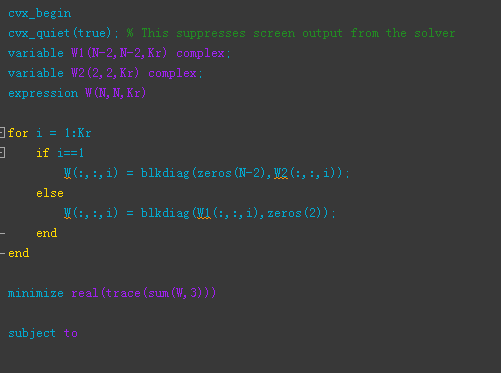# Assignment dimensions with subscripts do not match

%note：N：Number of antennas
% Kr:Number of users
%error:Assignment dimensions with subscripts do not match
% cvx/subsasgn (line 79)
% bx( :, ndx_x ) = by;
It seems to be due to an error caused by data type conversion. How to solve it?Is this error in a portion of the code which you didn’t show? You did not show the constraints.

Using CVX 2.1

``````N = 3
Kr = 2;
cvx_begin
variable W1(N-2,N-2,Kr) complex;
variable W2(2,2,Kr) complex;
expression W(N,N,Kr)

for i = 1:Kr
if i==1
W(:,:,i) = blkdiag(zeros(N-2)+ones(N-2)*1i,W2(:,:,i));
else
W(:,:,i) = blkdiag(W1(:,:,i),zeros(2)+ones(2)*1i); % error
end
end
``````

executed without warning or error message for me.

Also, rather than using an image, please copy and paste your code, then use the Preformatted text icon, as I did. This allows readers to copy and paste your code into their own MATLAB session.

1 Like

There is no error in the constraint department

``````cvx_begin
cvx_quiet(true); % This suppresses screen output from the solver
variable W1(N-2,N-2,Kr) complex;
variable W2(2,2,Kr) complex;
expression W(N,N,Kr)

for i = 1:Kr
if i==1
W(:,:,i) = blkdiag(zeros(N-2),W2(:,:,i));
else
W(:,:,i) = blkdiag(W1(:,:,i),zeros(2));
end
end

minimize real(trace(sum(W,3)))

subject to

for  k = 1:Kr
%Semi-definite formulation for the SINR constraint of user k
real(trace(W(:,:,k)*HH(:,:,k)))*(1+SINRconstraints(k))  >= SINRconstraints(k)*(1 + real(trace(sum(W,3)*HH(:,:,k))));
%Define the N x N beamforming matrix as Hermitian pos. semi-definite
W(:,:,k) == hermitian_semidefinite(N);
end
% Power constraints (L constraints)
for l = 1:L
real(trace(Q(:,:,l)*sum(W,3))) <= q(l);
end
cvx_end``````
1 Like
``````N = 10;
Kr = 3;
Q = zeros(N,N,N);
for j = 1:N
Q(j,j,j) = 1;
end
cvx_begin
cvx_quiet(true);
variable W1(N-2,N-2,Kr) complex;
variable W2(2,2,Kr) complex;
expression W(N,N,Kr)
for i = 1:Kr
if i==1
W(:,:,i) = blkdiag(zeros(N-2),W2(:,:,i));
else
W(:,:,i) = blkdiag(W1(:,:,i),zeros(2)); %error:Assignment dimensions with subscripts do not math  % cvx/subsasgn (line 79)  bx( :, ndx_x ) = by;
end
end

minimize real(trace(sum(W,3)))
subject to
for l = 1:L
real(trace(Q(:,:,l)*sum(W,3))) <= 20;
end
cvx_end``````

The constraints portions of your programs are not reproducible because you haven’t provided all the inputs. The code through the objective function executes without warning or error for me using CVX 2.1.

Which CVX version are you using? If you are using CVX 3.0beta, please try CVX 2.1. If that doesn’t solve the problem, then proceed to my next paragraph.

Last week, when answering another forum question, I encountered what appears to be a bug in which a CVX expression created using blkdiag didn’t behave consistently with the dimensions it supposedly had. The expression worked properly when I created the expression using “manual” MATLAB concatenation of the diagonal blocks with zero off-diagonal blocks. If you are encountering an error in a statement using blkdiag, as your comments seems to indicate, then I suggest you try forming by concatenation instead.

1 Like

thank you very much. I will give it a try.